## 3.20Other terms

In an equation for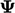, there can be terms other than the time derivative, advection and Laplacian of, including:

• a linear function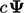where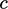is a scalar coeﬃcient or ﬁeld;
• a term without, sometimes including the derivative of another variable, e.g.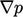.

The second example is simply discretised as an explicit gradient term as described in Sec. 3.15 . Derivative terms like this one are described in earlier sections and require no further discussion.

The ﬁrst example, the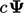term, requires much more discussion, particularly relating to the possibility of implicit discretisation. Let us consider the following equation: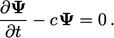(3.33)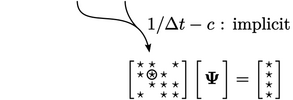Ifis discretised implicitly, then the matrix would contain a zero diagonal coeﬃcient when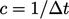, making it singular or non-invertible. If this occurs,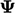is not present in the linear equation for the relevant cell, so it cannot be solved.

Therefore, such a term must be discretised explicitly when it has a negative sign (or positive on the right side of “=”), to ensure the matrix equation is solvable. The nature of Eq. (3.33 ) is thatcan only increase from an initial positive value.

### Implicit discretisation of linear terms

Let us now consider the equivalent equation with a linear term that has a positive sign, i.e.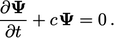(3.34)
With Eq. (3.34 ),can only decrease from an initial positive value but reaches a lower limit at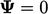. Like many scalar properties, e.g.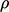,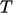,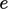, etc.,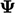may have a lower physical bound of 0, which the equation intentionally reﬂects.

It is important to maintain a physical bound of. Discretisation of Eq. (3.34 ) using the Euler time scheme Eq. (3.21 ) gives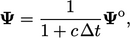(3.35)
which ensures boundedness since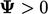. An equivalent explicit discretisation gives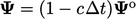which is only bounded when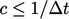, similar to the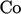limit imposed by explicit discretisation of advection, described in Sec. 3.17 . To avoid this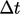limit, we apply implicit discretisation to terms with a positive sign on the left side of “=”, where possible.

Even when the term is not linear in, it can be implemented as such by “dividing and multiplying by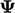”. For example, the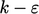turbulence model includes an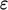term in the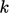equation, i.e.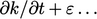, ignoring other terms. Dividing and multiplying theterm bygives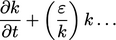(3.36)
producing a linear term inwhich can be discretised implicitly using a coeﬃcient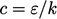.
Notes on CFD: General Principles - 3.20 Other terms